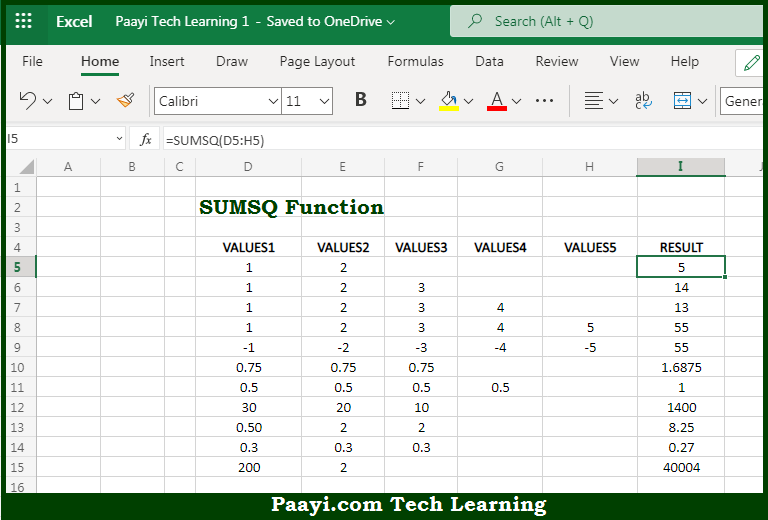# Learn How to Use Microsoft Excel SUMSQ Function

Written by | 0 Comments | 578 Views

In this article, you will learn how to use the Microsoft Excel SUMSQ function and its prime function in Microsoft Excel. You will also get to know the Microsoft Excel SUMSQ function return value and syntax with the help of some examples.

Microsoft Excel SUMSQ Function

The main function of the Microsoft Excel SUMSQ function is to get the squares of provided values. That implies, with the help of the SUMSQ function you can able to get the squares of values provided by you. It should be noted that the values that you provided can be in the form of constants, cell references, or ranges. Also keep in mind Empty cells, logical values, and text values are ignored when they appear in arrays or references. The logical values TRUE and FALSE are evaluated as 1 and 0 respectively, but only when they are hardcoded as arguments. Numbers entered as text that is "1", "3", etc., are evaluated, but only when they are hardcoded as arguments. So, with the help of the SUMSQ function, you can able to get the squares of provided values.

Return Value of SUMSQ Function

The return value will be the calculated sum of squares of values provided.

Syntax of SUMSQ Function

=SUMSQ(number1, [number2], ...)

Where the arguments:

• number1: This is the first argument or number containing numeric values.
• number2: This is the second argument or number containing numeric values (optional).

## How to Use Microsoft Excel SUMSQ Function?So we know that Microsoft Excel SUMSQ function you can able to get the squares of provided values. That implies, with the help of the SUMSQ function you can able to get the squares of values provided by you. It should be noted that the values that you provided can be in the form of constants, cell references, or ranges. So, with the help of the SUMSQ function, you can able to get the squares of provided values.